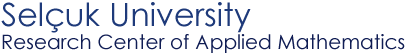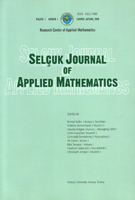Selçuk Journal of Applied Mathematics www.selcuk.edu.trSelçuk Journal of
Applied Mathematics

SPECIAL ISSUE

Summer-Autumn, 2001
Volume  2
Number 2

Research Center of
Applied Mathematics

 SJAM Summer-Autumn 2001, Volume 2 - Number 2 Continuity of numeric characteristics for asymptotic stability of solutions to linear difference equations with periodic coefficients Kemal Aydýn1, Haydar Bulgak1, Gennadii Demidenko2* 1 Research Centre of Applied Mathematics, Selçuk University, Konya, Turkey    email : hbulgak@selcuk.edu.tr  ; kaydin@selcuk.edu.tr 2 Sobolev Institute of Mathematics, SB RAS, Novosibirsk, Russia;   email: demidenk@math.nsc.ru Received: May 21, 2001 Summary.  We consider linear perturbation systems of difference equations y(n+1)=(A(n) + B(n))y(n), nł 0, where A(n), B(n) are N´N periodic matrices with period  T. The spectrum of a monodromy matrix of the system x(n+1)=A(n)x(n), nł 0 belongs to the unit disk |l| < 1. We indicate conditions on a perturbation matrix B(n)  for asymptotic stability of the zero solution to the perturbation system and prove continuity one numeric characteristic of the asymptotic stability from . Key words monodromy matrix, perturbation systems of difference equations, asymptotic stability of solutions. Mathematics Subject Classification (1991): 39A11 * The research was financially supported by the Scientific and Technical Research Council of Turkey (TUBITAK) in the framework of a NATO-PC Advanced Fellowships Programme. Article in PS format (86 kb) Article in ZIP format (34 kb)
 The article is available to read in  Ghostview format
 [Home] [contact]